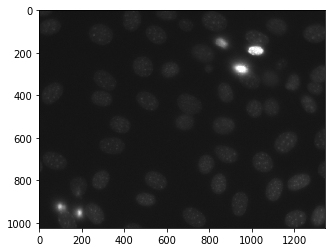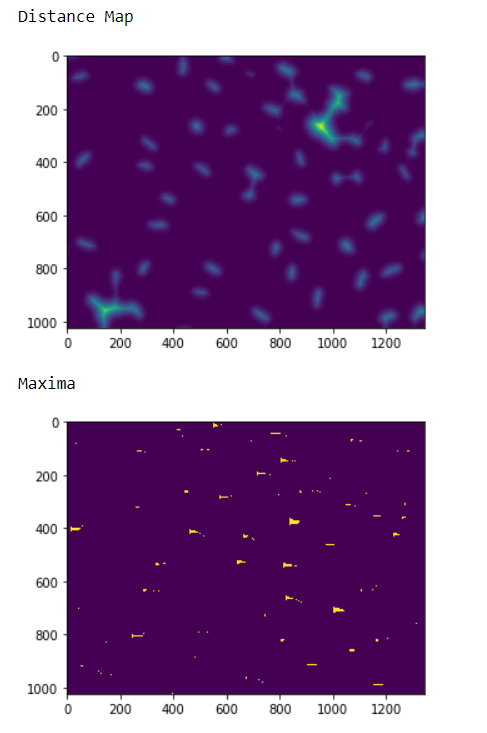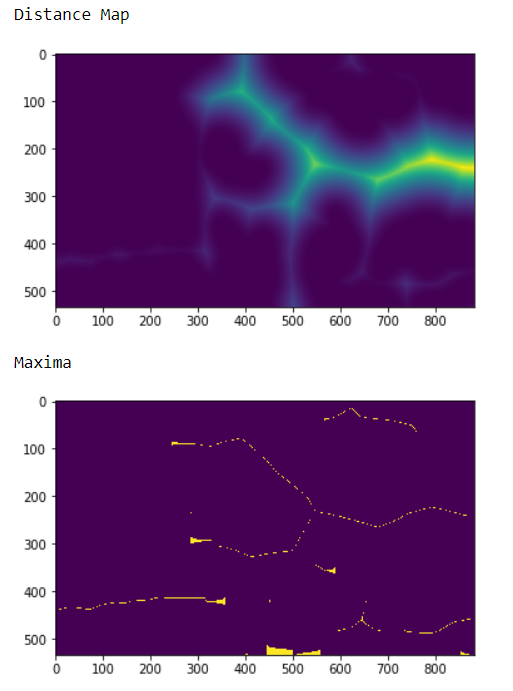# Mahotas – Highlighting Image Maxima

In this article we will see how we can highlight the maxima of image in mahotas. Maxima can be best found in the distance map image because in labeled image each label is maxima but in distance map maxima can be identified easily. For this we are going to use the fluorescent microscopy image from a nuclear segmentation benchmark. We can get the image with the help of command given below

`mahotas.demos.nuclear_image()`

Below is the nuclear_imageIn order to do this we will use `mahotas.morph.regmax` method

Syntax : mahotas.morph.regmax(img, Bc)

Argument : It takes image object and numpy ones array as argument

Return : It returns image object

Note : The input of the this should should be the filtered image or loaded as grey

In order to filter the image we will take the image object which is numpy.ndarray and filter it with the help of indexing, below is the command to do this

`iamge = image[:, :, 0]`

Example 1 :

 `# importing various libraries ` `import` `mahotas ` `import` `mahotas.demos ` `import` `mahotas as mh ` `import` `numpy as np ` `from` `pylab ``import` `imshow, show ` ` `  `# loading nuclear image ` `nuclear ``=` `mahotas.demos.nuclear_image() ` ` `  `# filtering iamge ` `nuclear ``=` `nuclear[:, :, ``0``] ` ` `  `# adding gaussian filter ` `nuclear ``=` `mahotas.gaussian_filter(nuclear, ``4``) ` ` `  `# setting threshold ` `threshed ``=` `(nuclear > nuclear.mean()) ` ` `  `# creating distance map ` `dmap ``=` `mahotas.distance(threshed) ` ` `  `print``(``"Distance Map"``) ` `# showing image ` `imshow(dmap) ` `show() ` ` `  `# numpy ones array ` `Bc ``=` `np.ones((``3``, ``2``)) ` ` `  `# getting maxima ` `maxima ``=` `mahotas.morph.regmax(dmap, Bc ``=` `Bc) ` ` `  `# showing image ` `print``(``"Maxima"``) ` `imshow(maxima) ` `show() `

Output :Example 2 :

 `# importing required libraries ` `import` `numpy as np ` `import` `mahotas ` `from` `pylab ``import` `imshow, show ` `  `  `# loading iamge ` `img ``=` `mahotas.imread(``'dog_image.png'``) ` `    `  `# filtering the imagwe ` `img ``=` `img[:, :, ``0``] ` `     `  `# setting gaussian filter ` `gaussian ``=` `mahotas.gaussian_filter(img, ``15``) ` `  `  `# setting threshold value ` `gaussian ``=` `(gaussian > gaussian.mean()) ` `  `  `# creating a labeled image ` `labeled, n_nucleus ``=` `mahotas.label(gaussian) ` `  `  ` `  `# getting distance map ` `dmap ``=` `mahotas.distance(labeled) ` ` `  `# showing image ` `print``(``"Distance Map"``) ` `imshow(dmap) ` `show() ` ` `  ` `  `# numpy ones array ` `Bc ``=` `np.ones((``4``, ``1``)) ` ` `  `# getting maxima ` `maxima ``=` `mahotas.morph.regmax(dmap, Bc ``=` `Bc) ` ` `  `# showing image ` `print``(``"Maxima"``) ` `imshow(maxima) ` `show() `

Output :Attention geek! Strengthen your foundations with the Python Programming Foundation Course and learn the basics.

To begin with, your interview preparations Enhance your Data Structures concepts with the Python DS Course.

My Personal Notes arrow_drop_upCheck out this Author's contributed articles.

If you like GeeksforGeeks and would like to contribute, you can also write an article using contribute.geeksforgeeks.org or mail your article to contribute@geeksforgeeks.org. See your article appearing on the GeeksforGeeks main page and help other Geeks.

Please Improve this article if you find anything incorrect by clicking on the "Improve Article" button below.

Article Tags :

1

Please write to us at contribute@geeksforgeeks.org to report any issue with the above content.